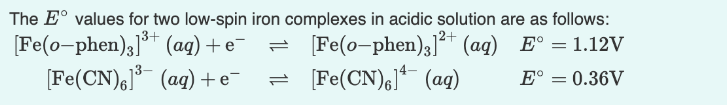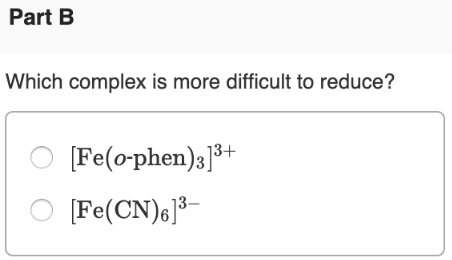# The E° values for two low-spin iron complexes in acidic solution are as follows: [Fe(-o-phen)3]^3+ (aq) + e^- ⇋ [Fe)o-phen)3]^2+ (aq) E° = 1.12V [Fe(CN)6]^3- (aq) + e^- ⇋ [Fe(CN)6]^4-(aq) E° = 0.36V Which complex is more difficult to reduce? a) [Fe(-o-phen)3]^3+ b) [Fe(CN)6]^3-